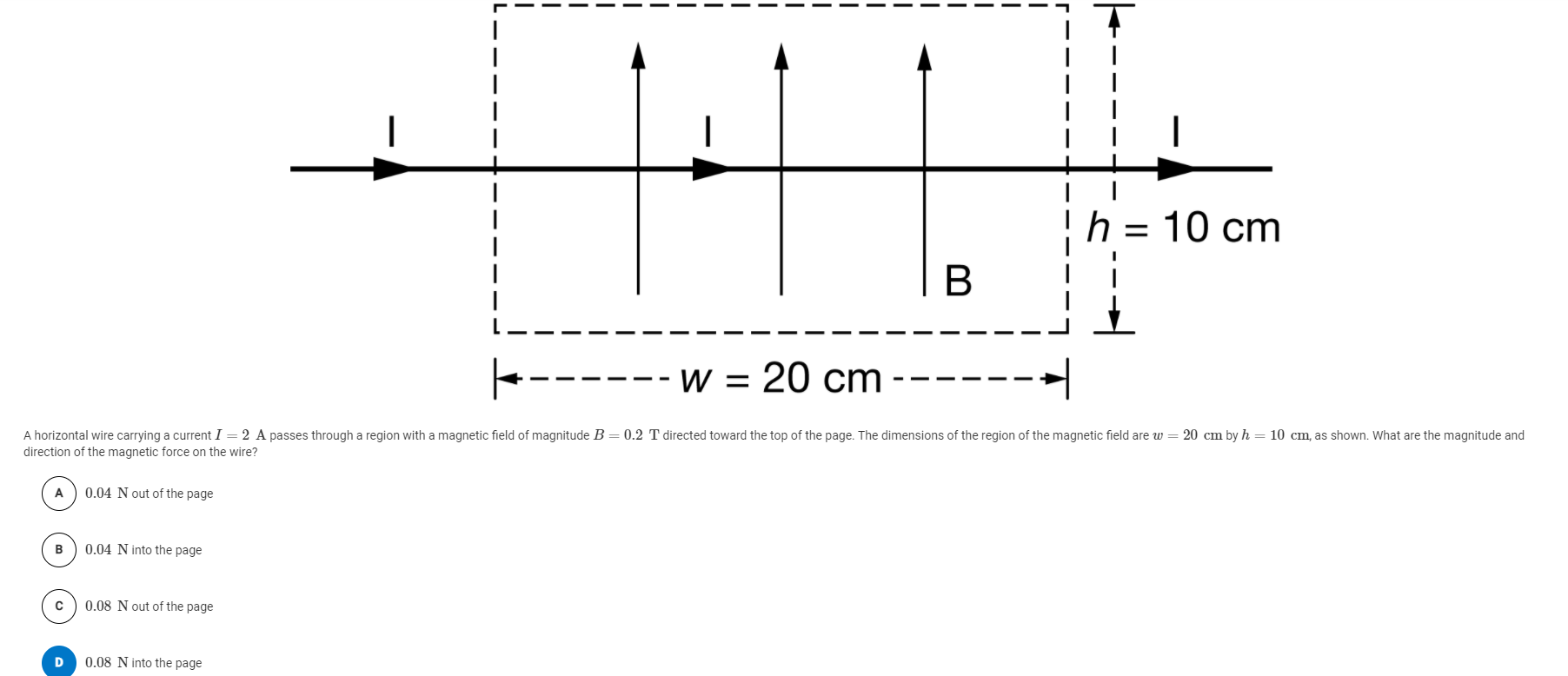# Question Solved1 AnswerAnswer choice E (not in screenshot): 8N, out of the page 1 1 h = 10 cm I I I B - W = 20 cm A horizontal wire carrying a current I = 2 A passes through a region with a magnetic field of magnitude B=0.2 T directed toward the top of the page. The dimensions of the region of the magnetic field are w = 20 cm by h = 10 cm, as shown. What are the magnitude and direction of the magnetic force on the wire? A 0.04 N out of the page B 0.04 N into the page с 0.08 N out of the page D 0.08 N into the pageAnswer choice E (not in screenshot): 8N, out of the page

Transcribed Image Text: 1 1 h = 10 cm I I I B - W = 20 cm A horizontal wire carrying a current I = 2 A passes through a region with a magnetic field of magnitude B=0.2 T directed toward the top of the page. The dimensions of the region of the magnetic field are w = 20 cm by h = 10 cm, as shown. What are the magnitude and direction of the magnetic force on the wire? A 0.04 N out of the page B 0.04 N into the page с 0.08 N out of the page D 0.08 N into the page
More
Transcribed Image Text: 1 1 h = 10 cm I I I B - W = 20 cm A horizontal wire carrying a current I = 2 A passes through a region with a magnetic field of magnitude B=0.2 T directed toward the top of the page. The dimensions of the region of the magnetic field are w = 20 cm by h = 10 cm, as shown. What are the magnitude and direction of the magnetic force on the wire? A 0.04 N out of the page B 0.04 N into the page с 0.08 N out of the page D 0.08 N into the page